# Disease Dynamics (SD)

This tutorial describes how to construct a model of an infectious disease. Before starting the tutorial, make sure you have familiarized yourself with how to create primitives and run models.
1. Create a new Stock named Healthy.
2. Create a new Stock named Infected.
3. The model diagram should now look something like this: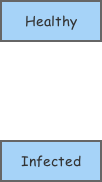4. Create a new Flow going from the primitive Healthy to the primitive Infected. Name that flow Infection.
5. The model diagram should now look something like this: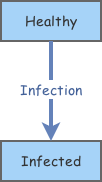The basic model structure has been laid-out, we can start to define parameter values and equations. We'll start with a very simple model containing a population of 100 people and where 2 people becoming sick each year.
6. Change the Initial Value of the primitive Healthy to 100.
7. Change the Flow Rate of the primitive Infection to 2.
8. Run the model. Here are sample results: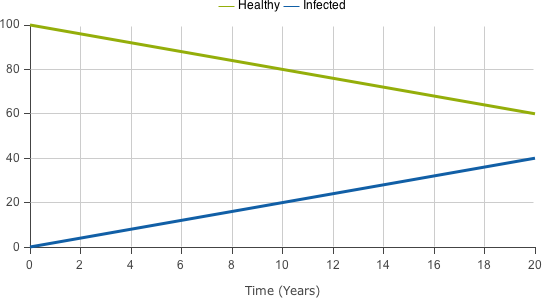The results are as we would expect. However this is not a model of an infectious disease as the infection rate does not depend on the presence of infected individuals. Let's change the infection rate so it depends on the contact rates between sick and healthy people.
9. Change the Flow Rate of the primitive Infection to 0.006*[Healthy]*[Infected].
10. Run the model. Here are sample results: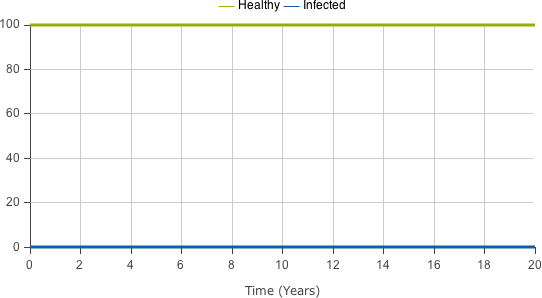That's strange. No one ever gets sick. Why is that? It turns out it is because we start the simulation with no infected people in the model. Since we're modeling an infectious disease, this means there is no one to start the epidemic! Let's change that we'll add a single infected person to get the epidemic started.
11. Change the Initial Value of the primitive Infected to 1.
12. Run the model. Here are sample results: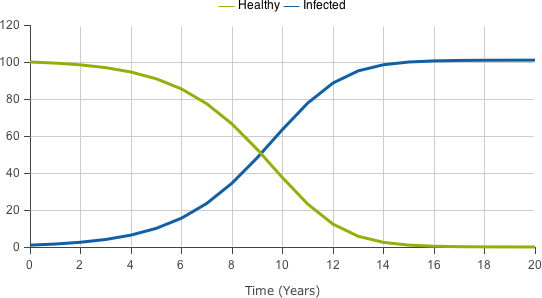That looks about right. Before moving on, let's spend a moment to improve our model structure. Right now if we wanted to edit the infection rate, we would have to dig down in the equations to find the right number. Let's make our model more modular, without changing any results, by separating the infection rate into its own variable.
13. Create a new Variable named Infection Rate.
14. Create a new Link going from the primitive Infection Rate to the primitive Infection.
15. The model diagram should now look something like this: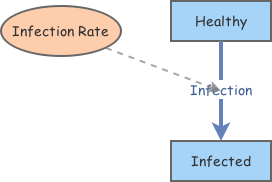16. Change the Equation of the primitive Infection Rate to 0.006.
17. Change the Flow Rate of the primitive Infection to [Infection Rate]*[Healthy]*[Infected].
18. Run the model. Here are sample results: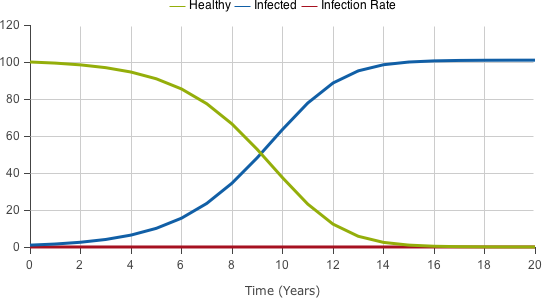We can hide the display of the infection rate primitive by configuring the display.
19. Run the model. Here are sample results: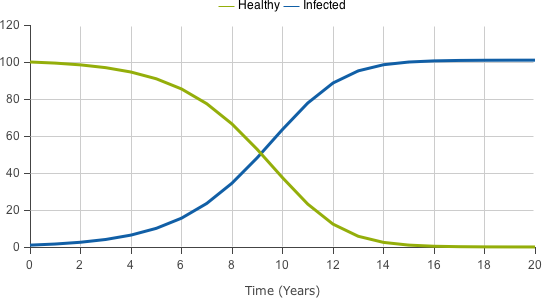Now that we have our basic model working, let's extend it by adding the phenomena of people recovering from the disease. We'll model something like the Chicken Pox where people become immune to the disease after they recover.
20. Create a new Stock named Immune.
21. Create a new Flow going from the primitive Infected to the primitive Immune. Name that flow Recovery.
22. Create a new Variable named Recovery Rate.
23. Create a new Link going from the primitive Recovery Rate to the primitive Recovery.
24. The model diagram should now look something like this: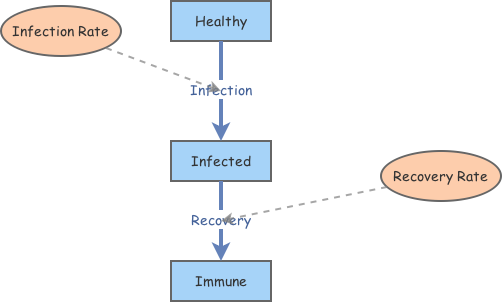25. Change the Equation of the primitive Recovery Rate to 0.1.
26. Change the Flow Rate of the primitive Recovery to [Recovery Rate]*[Infected].
27. Change the Equation of the primitive Infection Rate to 0.008.
28. Run the model. Here are sample results: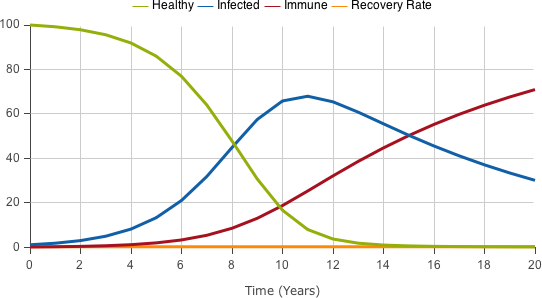Fantastic! Now we have a working disease simulation. You can experiment with different population sizes, infection rates and recovery rates to see how the results change.